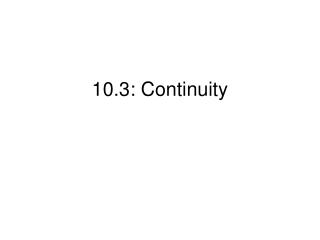DownloadDownload Presentation10.3: Continuity

# 10.3: Continuity

Download Presentation## 10.3: Continuity

- - - - - - - - - - - - - - - - - - - - - - - - - - - E N D - - - - - - - - - - - - - - - - - - - - - - - - - - -
##### Presentation Transcript

1. 10.3: Continuity

2. Definition of Continuity A function f is continuous at a pointx = c if 1. 2. f (c) exists 3. A function f is continuous on the open interval(a,b) if it is continuous at each point on the interval. If a function is not continuous, it is discontinuous.

3. A constant function is continuous for all x. • For integer n > 0, f (x) = xn is continuous for all x. • A polynomial function is continuous for all x. • A rational function is continuous for all x, except those values that make the denominator 0. • For n an odd positive integer, is continuous wherever f (x) is continuous. • For n an even positive integer, is continuous wherever f (x) is continuous and nonnegative.

4. Continuous at -1? Continuous at -2? YES NO Continuous at 2? YES Continuous at 0? Continuous at 2? YES NO Continuous at -4? YES

5. Discuss the continuity of each function at the indicated points F is continuous at -1 because the limit is 1 and also f(-1)=1 at x=-1 at x=0 Not continuous at 0 because g(0) is not defined at x=1 Not continuous at 1 because h(1) is not defined Not continues at 3 because k(3) is not defined and also the limit does not exist at x=3 and at x =0 Continuous at 0 because the limit is -1 and k(0)=-1